# Calculus 1 : Other Differential Functions

## Example Questions

### Example Question #11 : Other Differential Functions

What is the first derivative of f(x) = 2x * ln(sin(x))?

2tan(x)

2ln(sin(x))/cos(x)

2(ln(sin(x)) + x * tan(x))

2(ln(sin(x)) + x * cot(x))

2(ln(sin(x)) + x * cot(x))

Explanation:

f(x) = 2x * ln(sin(x))

Here, we have a product rule with a chain rule.

The derivative of ln(sin(x)) = (1/sin(x)) * cos(x). Note that this is cot(x).

Therefore, our full derivative is:

f'(x) = 2ln(sin(x)) + 2x * cot(x) = 2(ln(sin(x)) + x * cot(x))

### Example Question #12 : Other Differential Functions

What is the first derivative of f(x) = sin4(x) – cos3(x)?

4sin3(x) + 3cos2(x)

4sin3(x)cos(x) – 3cos2(x)sin(x)

sin4(x)cos(x) + cos3(x)sin(x)

sin(x) + 1

4sin3(x)cos(x) + 3cos2(x)sin(x)

4sin3(x)cos(x) + 3cos2(x)sin(x)

Explanation:

f(x) = sin4(x) – cos3(x)?

Treat these as chain rules:

The derivative of sin4(x) is therefore: 4sin3(x)cos(x)

The derivative of cos3(x) is therefore: 3cos2(x) * –sin(x) or –3cos2(x)sin(x)

This gives us 4sin3(x)cos(x) + 3cos2(x)sin(x), which really cannot be simplified any further.

### Example Question #13 : Other Differential Functions

What is the the first derivative of f(x) = 2tan(x)?

sec(x) * tan(x) * ln(2) * 2tan(x)

ln(2) * 2tan(x)

cot(x) * ln(2) * 2tan(x)

sec2(x) * ln(2) * 2tan(x)

ln(2) * 2sec(x)tan(x)

sec2(x) * ln(2) * 2tan(x)

Explanation:

This is a case of the chain rule.

Step 1: Deal with the exponential function

ln(2) * 2tan(x)

Step 2: Deal with the exponent

sec2(x)

sec2(x) * ln(2) * 2tan(x)

### Example Question #14 : Other Differential Functions

What is the first derivative of f(x) = sin4(x) – cos4(x)?

2sin(2x)

1

4

4sin3(x) + 4cos3(x)

4sin(4x)

2sin(2x)

Explanation:

Applying the chain rule to each element, we get:

f'(x) = 4sin3(x)cos(x) + 4cos3(x)sin(x)

If we factor out the common factors, we get:

f'(x) = 4sin(x)cos(x)(sin2(x) + cos2(x)) = 4sin(x)cos(x)(1) = 4sin(x)cos(x)

Also, since we know that 2sin(x)cos(x) = sin(2x), we know that 4sin(x)cos(x) = 2sin(2x)

### Example Question #15 : Other Differential Functions

What is the first derivative of f(x) = x2sin(4x3)?

2x * sin(4x3) + 12x5cos(4x3)

sin(4x3) + 12x2cos(4x3)

2x(sin(4x3) + 6x3cos(4x3))

2x * cos(4x3)

24x4 * cos(4x3)

2x(sin(4x3) + 6x3cos(4x3))

Explanation:

This is a product rule combined with a chain rule.  Let's do the chain rule for sin(4x3) first:

cos(4x3) * 12x2 = 12x2cos(4x3)

With this in mind, let's solve our whole problem:

2x * sin(4x3) + x2 * 12x2cos(4x3) = 2x * sin(4x3) + 12x4cos(4x3) = 2x(sin(4x3) + 6x3cos(4x3))

### Example Question #16 : Other Differential Functions

What is the first derivative of f(x) = x4 – x * sin(x–5)?

4x3 + cos(x–5)

4x3 – cos(x–5)

4x3 – sin(x–5) + (5 * cos(x–5)/x5)

4x3 + sin(x–5) – (5 * cos(x–5)/x5)

4x3 – sin(x–5) + (5 * cos(x–5)/x5)

Explanation:

The first element is merely differentiated as 4x3

The second element is a relatively simple product rule:

sin(x–5) + x * cos(x–5) * –5 * x–6 = sin(x–5) + cos(x–5) * –5 * x–5 = sin(x–5) – (5 * cos(x–5)/x5)

Put everything back together:

4x3 – ( sin(x–5) – (5 * cos(x–5)/x5) ) = 4x3 – sin(x–5) + (5 * cos(x–5)/x5)

### Example Question #11 : Other Differential Functions

What is the first derivative of f(x) = 5x * ln(2x)?

5(ln(2) + (1/2))

5(ln(2) + 1)

5/x

5/2x

5ln(2)/2x

5(ln(2) + 1)

Explanation:

This is just a normal product rule problem:

5 * ln(2x) + 5x * 2 * (1/2x)

Simplify: 5ln(2) + 5 = 5(ln(2) + 1)

### Example Question #15 : Other Differential Functions

What is the slope of the tangent line at x = 2 for f(x) = 6/x2?

–1.5

1.5

3

–12

–3

–1.5

Explanation:

First rewrite your function to make this easier:

f(x) = 6/x= 6x–2

Now, we must find the first derivative:

f'(x) = –2 * 6 * x–3 = –12/x3

The slope of the tangent line of f(x) at x = 2 is: f'(2) = –12/23 = –12/8 = –3/2 = –1.5

### Example Question #16 : How To Find Differential Functions

What is the first derivative of f(x) = 2ln(cos(x)sin(x))?

2cot(x)cos2(x)

cot(x)cos2(x)

2cos2(x)sec(x)csc(x)

2cos(2x)sec(x)csc(x)

2cos(2x)sec(x)csc(x)

Explanation:

This requires both the use of the chain rule and the product rule. Start with the natural logarithm: 2/(cos(x)sin(x))

Now, multiply by d/dx cos(x)sin(x), which is: –sin(x)sin(x) + cos(x)cos(x) = cos2(x) – sin2(x)

Therefore, f'(x) = (cos2(x) – sin2(x)) * 2/(cos(x)sin(x)) = 2(cos2(x) – sin2(x))sec(x)csc(x)

From our trigonometric identities, we know cos2(x) – sin2(x) = cos(2x)

Therefore, we can finilize our simplification to f'(x) = 2cos(2x)sec(x)csc(x)

### Example Question #11 : Other Differential Functions

Take the derivative of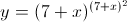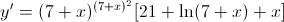Derivative does not exist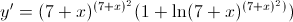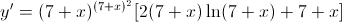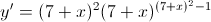Explanation:

We need to use logarithm differentiation to do this problem.  Take the natural log of both sides.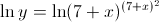Apply the power rule of natural log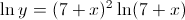Perform implicit differentiation to both sides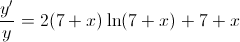Solve for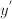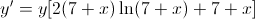### All Calculus 1 Resources